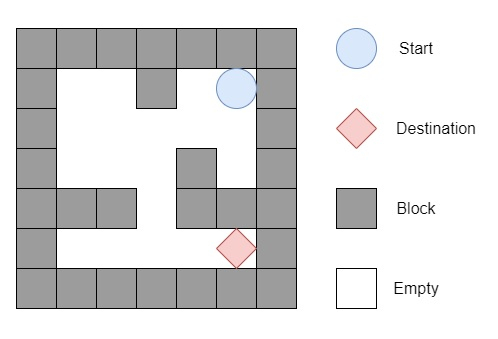# The Maze II in C++

Suppose there is a ball in a maze with empty spaces and walls. Now the ball can go through empty paths by rolling any direction like up, down, left or right, but it won't stop rolling until hitting a wall. When the ball stops, it could choose the next direction.

We have to start position of ball, the destination and the maze, we have to find the shortest distance for the ball to stop at the destination. Here the distance is actually defined by the number of empty cells, that are covered by the ball (Excluding start position, including starting position). If that is impossible to stop the ball at destination, then return -1.

The maze is represented by one 2D array. Here 1 indicates the wall and 0 indicates the empty space. The borders of the maze are all walls. The start and destination coordinates are represented by row and column indices.

So, if the input is like a maze represented by a 2D array

 0 0 1 0 0 0 0 0 0 0 0 0 0 1 0 1 1 0 1 1 0 0 0 0 0

start position is (0, 4) destination position is (4, 4), then the output will be 12, One possible way is : left to down to left to down to right to down to right. (1+1+3+1+2+2+2) = 12To solve this, we will follow these steps −

• n := row count, m := column count

• ret := infinity

• Define one 2D array dist of order n x m

• Define one queue q

• insert start into q

• dist[start, start] := 0

• while (not q is empty), do −

• curr := first element of q

• delete element from q

• x := curr, y := curr

• if x is same as destination and y is same as destination, then −

• ret := minimum of ret and dist[x, y]

• currDist := dist[x, y]

• tempDist := 0

• i := x

• while (i + 1 < n and grid[i + 1, y] is zero), do −

• (increase i by 1)

• (increase tempDist by 1)

• if currDist + tempDist < dist[i, y], then −

• dist[i, y] := currDist + tempDist

• insert { i, y } into q

• i := x

• tempDist := 0

• while (i - 1 >= 0 and grid[i - 1, y] is zero), do −

• (increase tempDist by 1)

• (decrease i by 1)

• if currDist + tempDist *lt; dist[i, y], then −

• dist[i, y] := currDist + tempDist

• insert { i, y } into q

• i := y

• tempDist := 0

• while (i - 1 >= 0 and grid[x, i - 1] is zero), do −

• (decrease i by 1)

• (increase tempDist by 1)

• if currDist + tempDist < dist[x, i], then −

• dist[x, i] := currDist + tempDist

• insert { x, i } into q

• i := y

• tempDist := 0

• while (i + 1 < m and grid[x, i + 1] is zero), do −

• (increase i by 1)

• (increase tempDist by 1)

• if currDist + tempDist < dist[x, i], then −

• dist[x, i] := currDist + tempDist

• insert { x, i } into q

• return (if ret is same as inf, then -1, otherwise ret)

## Example

Let us see the following implementation to get better understanding −

Live Demo

#include <bits/stdc++.h>
using namespace std;
class Solution {
public:
int shortestDistance(vector<vector<int<>& grid, vector<int<& start, vector<int<& destination) {
int n = grid.size();
int m = n? grid.size() : 0;
int ret = INT_MAX;
vector < vector <int< > dist(n, vector <int<(m, INT_MAX));
queue < vector <int< > q;
q.push(start);
dist[start][start] = 0;
while(!q.empty()){
vector <int< curr = q.front();
q.pop();
int x = curr;
int y = curr;
if(x == destination && y == destination){
ret = min(ret, dist[x][y]);
}
int currDist = dist[x][y];
int tempDist = 0;
int i = x;
while(i + 1 < n && !grid[i + 1][y]){
i++;
tempDist++;
}
if(currDist + tempDist < dist[i][y]){
dist[i][y] = currDist + tempDist;
q.push({i, y});
}
i = x;
tempDist = 0;
while(i - 1 >= 0 && !grid[i - 1][y]){
tempDist++;
i--;
}
if(currDist + tempDist < dist[i][y]){
dist[i][y] = currDist + tempDist;
q.push({i, y});
}
i = y;
tempDist = 0;
while(i - 1 >= 0 && !grid[x][i - 1]){
i--;
tempDist++;
}
if(currDist + tempDist < dist[x][i]){
dist[x][i] = currDist + tempDist;
q.push({x, i});
}
i = y;
tempDist = 0;
while(i + 1 < m && !grid[x][i + 1]){
i++;
tempDist++;
}
if(currDist + tempDist < dist[x][i]){
dist[x][i] = currDist + tempDist;
q.push({x, i});
}
}
return ret == INT_MAX ? - 1 : ret;
};
main(){
Solution ob;
vector<vector<int<> v = {{0,0,1,0,0},{0,0,0,0,0},{0,0,0,1,0},{1,1,0,1,1},{0,0,0,0,0}};
vector<int< v1 = {0,4}, v2 = {4,4};
cout << (ob.shortestDistance(v, v1, v2));
}

## Input

{{0,0,1,0,0},{0,0,0,0,0},{0,0,0,1,0},{1,1,0,1,1},{0,0,0,0,0}}, {0,4},{4,4}

## Output

12

Updated on: 19-Nov-2020

344 Views